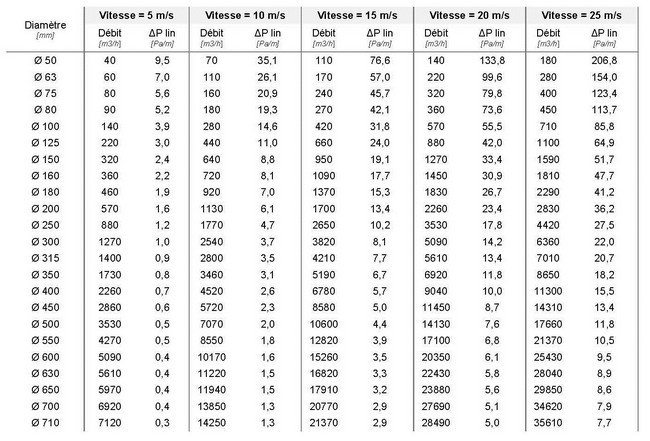## 1. DEFINITION

Pressure drops represent the total pressure drop due to the various inevitable frictions suffered by the flowing air and are expressed in Pascals.

The pressure drops are directly related to the speed of the fluid and there are two types of pressure drop:
-Linear or regular pressure drops due to air friction on the walls of the pipes.
-Singular pressure drops due to the different singularities of the network (air inlets and outlets, elbows, reductions, etc.).
The total pressure drop is the sum of the linear and singular drops.

## 2. ESTIMATED LINEAR PRESSURE DROP

The table below shows the linear pressure drop in Pascal/meter as a function of conveying speed and pipe diameter. The flow rates indicated in the table are rounded values.

The pressure drop values are determined from the formula in paragraph 3 — Calculation formulas — and correspond to a linear pressure drop for air at 20°C with smooth pipes.## 3. FORMULAS

The linear pressure loss is expressed as:
ΔP linear = (L. λ. ρ. V²) / ( 2. Ø) [Pa] [Pa]

with

λ: Linear pressure drop coefficient

ρ: Density of the fluid in [kg/m3].

V: Fluid velocity in [m/s].

Ø: Diameter in [m]

L: Length of the pipe [m]

The expression for the singular pressure drop is:
ΔP singular = 0.5. ρ. K. V² [Pa]

with

λ: Linear pressure drop coefficient

ρ: Density of the fluid in [kg/m3].

V: Fluid velocity in [m/s].

Total pressure drop = Ʃ ΔP linear + Ʃ ΔP singular